﻿ Monitoring and Modeling of Chlorophyll-a Dynamics in a Eutrophic Lake: M'koa Lake (Jacqueville, Ivory Coast)Publications are Open
Access in this journal
Article Versions
Export Article
• Normal Style
• MLA Style
• APA Style
• Chicago Style
Research Article
Open Access Peer-reviewed

### Monitoring and Modeling of Chlorophyll-a Dynamics in a Eutrophic Lake: M'koa Lake (Jacqueville, Ivory Coast)

Yapo Habib Kpidi, Ossey Bernard Yapo, Mamadou Guy-Richard Koné , Gabaze André Gadji, Agness Essoh Jean Eudes Yves Gnagne, Jean Stéphane N’dri, Nahossé Ziao
American Journal of Environmental Protection. 2018, 6(1), 1-9. DOI: 10.12691/env-6-1-1
Published online: January 09, 2018

### Abstract

In-situ measurements and physico-chemical analyzes of thirty (30) samples taken bimonthly from August 2015 to December 2016 on six (6) stations of Lake M'koa were carried out. A modeling study was made in order to determine a quantitative and qualitative relationship between chlorophyll-a and five physico-chemical descriptors (temperature, turbidity, oxidative power (RH), nitrate ions (NO3-) and nitrite (NO2-)). These descriptors constituted the explanatory and predictive parameters of chlorophyll-a of samples taken from Lake M'koa. This study was carried out by using Principal Component Analysis (PCA), Ascending Hierarchical Classification (AHC), Multiple Linear Regression (RML) and Nonlinear (RMNL) methods. Two quantitative and qualitative linear and nonlinear models (RML and RMNL) have been proposed. These accredited models as good statistical indicators have been validated according to the rules established by the Organization of Economic Cooperation and Development (OECD). Statistical indicators of RMNL reveal more efficient predictions with R2 = 0.942, RMSE = 0.049 and F = 291.986. The obtained results suggest that the combination of these five descriptors could be useful in predicting the property of chlorophyll-a. In addition, turbidity is the first most important descriptor for the prediction of chlorophyll-a at the M'koa Lake different stations.

### 1. Introduction

The quality of surface water is one of the harmful environmental difficulties facing humanity 1, 2 The undermining of these waters of surface quality can be due to agricultural activities, industrial and domestic waste ejected into the receiving environment without any treatment. Significant efforts have been made in recent decades to develop water management strategies in order to ensure good quality of water 3. The quality of water is also one of the main cause of most public health problems in developing countries 4, 5. The classical approach to assess the trophic state of a given area consists of physico-chemical and biological measurements of a group of environmental variables, which is depending on several constraints. Nowadays, there is a growing interest concerning the development of alternative methods for the monitoring and rational management of water resources. Eutrophication is defined as a process of natural aging of aquatic environments, which is accelerated by human beings activities. These human beings activities can be industrial effluents and/or domestic wastewater discharged without treatment or partially treated 6 in aquatic systems. The rejections are all riche in nitrogen and phosphorus and will enriched the aquatic area. These nutritive salts considered as trophic indicators 7 are likely to favor the appearance of algae and macrophytes whose growth is often linked to seasonal variations and various environmental factors 8, 9. Many environmental factors such as temperature, pH and dissolved oxygen content must also be considered. Indeed, the solubility of oxygen in water is inversely proportional to its temperature. The temperature (25 to 30°C average in our tropics), the concentration of dissolved oxygen and organic matter are physico-chemical factors that govern a lot of chemical and biological processes taking place in water 10. The existence of various activities on the watershed can contribute to the degradation of the quality of its aquatic system 11. To this end, a continuous supervision of its physico-chemical and biological quality, a deep knowledge of its hydro dynamism and different factors of disturbance of the equilibrium of this lacustrine ecosystem must be well-known 12. Physico-chemical and biological approaches to eutrophication allowed to quantify the trophic state of a given area by measuring environmental variables and compared them to established norms. This approach seems to be expensive in terms of equipment and reagents. to overcome the considerable logistical, chemical and investments requirement d for the occasional or continuous sampling in order to the characterize of the aquatic environment 13, the development of alternative methods for the monitoring and the rational management of resources in waters constituted a real godsend., For the fulfillment of this aim, the use of modeling remains a way of approach. Indeed, dissolved oxygen have been increasingly modeled in the assessment of the health status of an aquatic area by using a statistical approach 14. It appears necessary to model chlorophyll-a because it seems to express the environmental response to ecological disturbances and to circumscribe them. Thus, a Quantitative Structure Property Study (QSPR) is used to determine elaborated models from physico-chemical descriptors. This statistical approach can combine methods such as linear regression, nonlinear regression, and so on.

The general objective of this work is to design statistical models by using multiple regressions (linear and nonlinear) which will be able to predict the chlorophyllian property of M'koa Lake’s water from environmental variables. Specifically, this involves identifying pertinent and expressive chlorophyll-a explanatory variables in order to develop simulation tools that will be useful for integrated and sustainable management of water resources.

### 2. Materials and Methods

2.1. Presentation of the Study Area

Situated in the region of large bridges (Grands Ponts), 62 km west of Abidjan, Lake M'koa is an endorheic lake without surface outfall, in Jacqueville (Côte d'Ivoire), constitutes the framework of this study (Figure 1). A shallow natural lake with a maximum depth of 3.9 m and an area of about 0.180 km2, Lake M'koa, in the sub-equatorial Attiean climate, is maintained by infiltration 10.

• Figure 1. Mapping of the study area
2.2. Physico-Chemical Descriptors

Chlorophyll-a is the main form of chlorophyll present in organisms that carry out photosynthesis. It is the pigment present in almost all plants and captures the light necessary for photosynthesis. This process is very important because it allows plants to transform carbon dioxide (CO2) into organic matter. Turbidity is a strong indicator for wetland monitoring and planning. Water quality supervising using modeling is essential for mapping turbidity in water 15. This indicator is a useful one which allows to predict the state of health of the wetland in order to maintain its ecosystem healthy 16. Ammonia (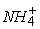), nitrite () and nitrate (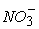), which are the inorganic nitrogenous forms, are common pollutants in water of surface and groundwater. These inorganic forms are causing enormous health problems on humans and animals 17, 18. The oxidizing power (RH) permits to determine the oxidizing or reducing character of the sampled water evaluated as follows: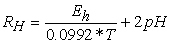(1)

Eh: average redox potential; T: temperature in kelvin degree (273 + T ° C).

The value of the oxidizing power allows to classify waters in the following categories according to Rejset 19:

- RH ≥ 23, the medium is called oxidizing;

- 15 < RH <23, the medium is described as anoxic;

- RH <15, the medium is said to be reducing.

These different physico-chemical descriptors were determined by kpidi et al. 10. The modeling was done by using the multilinear and nonlinear regression method implemented in Excel spreadsheets 20 and XLSTAT version 2014 21.

2.3. Estimation of the Predictive Capacity of a Model

Chlorophyll-a from thirty (30) studied samples showed significant variation in concentrations (17.16 to 72.8 μg/L). For the fulfilment of our aim, a log-based ten-fold transformation of the activity was applied to obtain higher mathematical values when the structures are biologically very efficient 22, 23. Biological properties are generally estimated by the opposite of the logarithm. Chlorophyll-a expressed by its potential (pChl-a) which is defined by equation (2):(2)

Thus, this range of reduced variation of concentrations permit to define a better quantitative relationship between the chlorophyll-a and the physico-chemical descriptors of the taken samples. The quality of a model is determined by taking care with various statistical analysis indicators including the coefficient of determination R2, the standard error (RMSE), the correlation coefficients of the cross validationand Fischer F. R2 , RMSE and F relate to the adjustment of calculated and experimental values. They describe the predictive power within the model limits and allow to express the accuracy of the calculated values on the test set 24, 25. As for the cross-validation coefficient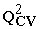, it gives information on the predictive power of the model, which can be "internal" because it is calculated from the structures used to construct this model. The coefficient of determination R² gives an evaluation of the dispersion of the calculated values around the experimental ones. The quality of the modeling is better when the points evaluated by the coefficient R² are close to the line of adjustment 26. R² is expressed as follows:(3)

Or: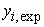: Experimental value of chlorophyll-a,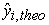: Calculated value of chlorophyll-a and: Experimental average value of chlorophyll-a

The closer the value of R² is to 1, the better the computed and experimental values are correlated. Moreover, the varianceis determined by the relation (4)(4)

Where k is the number of independent variables (descriptors), n is the observation number of the test or training set and n-k-1 is the degree of freedom.

The standard error or standard deviation RMSE is another statistical used indicator. It allows to evaluate the reliability and the accuracy of a model:(5)

The Fisher F test is also used to measure the level of statistical significance of the model, in other words the quality of the choice of descriptors constituting the model.(6)

The coefficient of determination of the cross-validationwhich makes it possible to evaluate the accuracy of the prediction on the test set is determined by means of the following relation:(7)
2.4. Statistical Analyzes

Principal Component Analysis (PCA) is a data analysis tool that can be used to explain the structure of correlations or covariances using linear combinations of original data. Its use permit to interpret the data in a reduced space 27. It was used to appreciate, certainly, the relations between the different measured variables, above all to access their structuring in order to be able to gather them by zone. The fact of grouping them per zone thus meets the objective of the slighted approach, which is to correlate the classes of physico-chemical descriptors obtained during the sampling.

The Ascending Hierarchical Classification (AHC) aims to partition into homogeneous classes a set of individuals (an individual is an observation and, in our case, they are samples) 28. It organizes individuals, defined by a number of variables and modalities, by grouping them hierarchically on a dendrogram. It aggregates those that are most similar by using measures of dissimilarity or distance between individuals to form classes. It is made from the data of individuals and variables. AHC allowed to establish a typology of the samples according to the temperature, turbidity, RH, NO3- and NO2-.

The Multiple Linear Regression (RML) statistical technique is used to study the relationship between a dependent variable (Property) and several independent variables (descriptors). This statistical method minimizes the differences between the actual and predicted values. It also allowed to select the descriptors used as input parameters in multiple nonlinear regression (RMNL). As for the analysis of nonlinear multiple regression (RMNL), it is also used for betterment of the structure-property relationship in order to evaluate quantitatively the property. It is the most common tool for studying multidimensional data. It is based on the following XLSTAT preprogrammed functions:(8)

Where a, b, c, d, ... represent the parameters and, x1, x2, x3, x4,... represent the variables. RML and RMNL were generated using the XLSTAT software version 2014 21, to predict chlorophyll-a. The equations of the different models were evaluated by the coefficient, the mean squared error (RMSE), the Fischer test (F) and the cross correlation coefficient (Q2CV) 29.

2.5. Criterion of Acceptance of a Model

The performance of a mathematical model, for Eriksson et al. 30, is characterized by a value offor a satisfactory model and for an excellent model,. According to Tropsha et al. 31, 32, 33, for the external validation set, the predictive power of a model can be obtained from the following five criteria

1)2)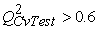3)4)and,

5)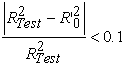and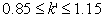.

Roy and Roy 34 have further refined the predictive skill of a model. They developed quantities such as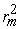and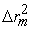, called metric values.determines the proximity between the observed activities and the predicted ones. The metric valuesandare calculated from the observed and predicted activities. Currently, these two different variantsandcan be calculated for the test set (internal validation) or for the test set (external validation). A model is acceptable for these authors, if both of these criteria are met.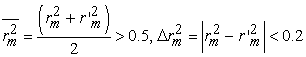Where: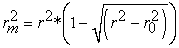and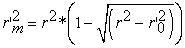.

### 3. Results and Discussion

The set of descriptor values for twenty (20) samples of the test set and ten (10) other samples of the validation set are presented in Table 1.

3.1. Typology of the Waters of Lake M'koa

The Pearson (n) correlation matrix (Table 2), the correlation circle (Figure 2), the Cartesian diagrams according to F1 and F2 (Figure 3 and Figure 4) and the dendrograms of the stations are shown below.

The PCA data matrix gathers the average values of five (5) variables representing physico-chemical descriptors, and thirty (30) individuals representing the original samples. The resulting matrix provides information on the negative or positive correlation between the variables. Turbidity is positively correlated with pChl-a (r = 0.7094 and p <0.05) at a significant level. The examination of the community circle of Figure 2 associated with the analysis of the PCA factor structure (Table 1), indicate two (02) main components represented by F1 and F2 which corresponds respectively to 33.33% and 29.36%, all explained variance. These two factors totalizing 62.69% of the total variance are sufficient to interpret the entire PCA data. In fact, high temperature and turbidity are positively correlated with factor 1. Conversely, pChl-a is negatively and moderately correlated. Factor 1 reflects a pollution gradient. Concerning factor 2, NO2- and NO3-, which participate to the synthesis of chlorophyll-a, are strongly and positively correlated with the opposite of RH, which is negatively correlated with factor 2. Factor 2 seems to indicate an increasing gradient of oxidation.

• Figure 2. Correlation circle of physico-chemical descriptors according to F1 x F2
• Figure 3. Cartesian diagram according to F1 and F2: correlation between the descriptors used and chlorophyll-a
• Figure 4. Cartesian diagram according to F1 and F2
• Figure 5. Dendrograms of the samples

The Cartesian diagram of Figure 3 shows that the sample 28 is rich in inorganic nitrogen (NO2- and NO3-) when the samples 5, 10 and 22 with a high oxidizing power are inversely proportional to the first one. At the same time, samples 14, 18, 19 and 25 have high turbidity and temperature.

Figure 4 illustrates a sample distribution in three classes (C1, C2 and C3) characterizing the physico-chemical descriptors. Thus, the class C1 characterizes the pollution marked by the turbidity and the temperature while the class C2 is marked by the inorganic nitrogen compounds (NO2- and NO3-) and finally the class C3 marked by RH. This classification is corroborated by the Ascending Hierarchical Classification which also highlights these three groups of samples.

3.2. Multiple Linear Regression (RML)

The equation of the RML model with these statistical indicators is presented below. Figure 6 shows the fitting right of the experimental and theoretical data of pChl-a test sets (blue dots) and validation (red dots) of the model. This obtained model relates the Chlorophyll-a property and the physico-chemical descriptors of the samples used. The negative or positive sign of the coefficient of a descriptor of model reflects the effect of proportionality in the evolution of chlorophyll-a and this parameter of the regression equation. The negative sign indicates an inversely proportional evolution between Chlorophyll-a and this descriptor. The positive sign reflects the opposite effect. The equation obtained is presented below:(9)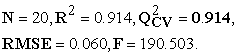The negative signs of the RH coefficients, turbidity and NO2-indicate that these descriptors contribute more to the synthesis of chlorophyll-a in the aquatic environment. Temperature and concentration of NO3- which are positive descriptors of the regression equation appear to contribute to the increasing production of chlorophyll-a. The significance of the model is shown by the high value of the Fischer coefficient which is F = 190.503. In addition, the robustness of this model is translated by the correlation coefficient of the cross validation= 0.914 > 0.9. The regression line established by the experimental and theoretical data of pChl-a test and validation sets is shown in Figure 6.

The low value of the standard error RMSE = 0.060 attests to the good similarity between the predicted and experimental values (Figure 7). This curve shows a similar evolution of these data by the multilinear model pChl-a of Lake M'koa, despite some recorded differences.

• Figure 6. Regression line of the test and validation phases of the RML model
• Figure 7. Similarity curve of the experimental and predicted values of the RML model

Verification of Tropsha’s Criteria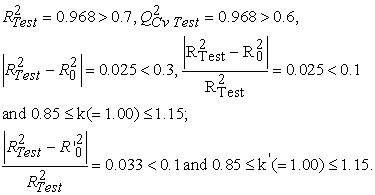All values meet the Tropsha criteria, so the model is acceptable for the prediction of M'koa Lake chlorophyll-a.

3.3. Nonlinear Multiple Regression (RMNL)

The statistical nonlinear regression method was used to improve quantitatively the prediction of pChl-a. It takes into account the five selected descriptors (Temperature, RH, Turbidity, NO2-, NO3-). It is the most common tool for studying multidimensional data. This statistical method is applied to the data in Table 1 for thirty (30) samples associated with the five (5) physico-chemical descriptors. The resulting equation is expressed as follows:(10)The significance of the model is defined by the Fischer coefficient F = 291.986. Concerning the robustness of this model, the correlation coefficient of the cross validation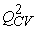= 0,942 > 0.9, the model is said to be excellent. The line of this model is illustrated in Figure 8.

The very low value of the standard error, RMSE = 0.049, also demonstrates the good similarity between predicted and experimental values (Figure 9). This curve reflects a very good analogue evolution of the experimental values predicted by the Lake M'koa RMNL model, despite some discrepancies.

Verification of Roy's Criteriawhich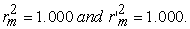The two metric criteria of Roy et al. are verified, the model is therefore acceptable for the prediction of chlorophyll-a at M'koa Lake. On both models, the model obtained by the RMNL statistical method has a much better predictive ability. However, since this model is a function of five physico-chemical descriptors, it is essential to determine the contribution of each of them in the prediction of the chlorophyll-a property. Indeed, the knowledge of this contribution permits to establish the order of priority of the various descriptors and to define the choice of the parameters to be optimized for the good prediction and understanding of the eutrophication of the M'koa lake.

• Figure 8. Regression line of the RMNL test and validation phases
• Figure 9. Similarity curve of the experimental and predicted values of the RMNL model
• Figure 10. Contribution of physico-chemical descriptors in models
3.4. Analysis of the Contribution of the Descriptors

The contribution of the five physico-chemical descriptors in the prediction of chlorophyll-a series of samples was determined from the XLSTAT version 2014 software 21. The different contributions are illustrated in Figure 10.

The order of priority of physico-chemical descriptors is classified according to the following sequence: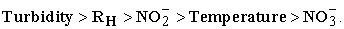According to this sequence, turbidity displays a high proportion (-0.5743) and nitrate with 0.2140 the lowest proportion compared to other descriptors. It should be noted that turbidity is the most influential physico-chemical descriptor. Thus, to reduce the phytoplankton biomass of Lake M'koa, it is necessary to reduce the turbidity as much as possible. This means reducing or avoiding any activities that generate particular pollution in Lake M'koa.

### 4. Conclusion

This study allows to highlight relationships between chlorophyll-a, which is a fundamental property of phytoplankton manifestation, and the physico-chemical descriptors measured in the samples. The physico-chemical descriptors (Turbidity, RH, NO2-, Temperature, NO3-) permit to explain and predict the property of chlorophyll-a because there are strong correlations between the calculated and experimental values. The multivariate analysis allowed us to detect three classes, namely class C1 characterizing the organic pollution expressed by turbidity and temperature. Class C2, which is characterized by inorganic nitrogen pollution (NO2- and NO3-) and finally class C3 expressed by RH. The study of the robustness of the two models (RML and RMNL) presented a good stability and an excellent power of prediction. In addition, the RMNL model (R2 = 0.942, RMSE = 0.049, F = 291.986) is better and is an effective tool for predicting chlorophyll-a at M'koa Lake. Moreover, the study of the contribution of physico-chemical descriptors has shown that turbidity is the priority descriptor in the prediction of chlorophyll-a.

### References

Published with license by Science and Education Publishing, Copyright © 2018 Yapo Habib Kpidi, Ossey Bernard Yapo, Mamadou Guy-Richard Koné, Gabaze André Gadji, Agness Essoh Jean Eudes Yves Gnagne, Jean Stéphane N’dri and Nahossé ZiaoThis work is licensed under a Creative Commons Attribution 4.0 International License. To view a copy of this license, visit http://creativecommons.org/licenses/by/4.0/